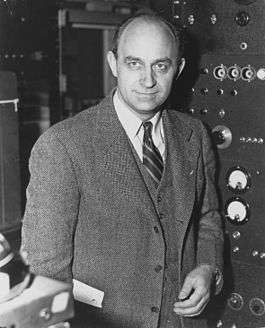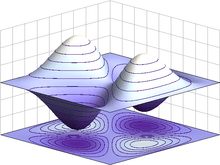FermionEnrico FermiAntisymmetric wavefunction for a (fermionic) 2-particle state in an infinite square well potential.

In particle physics, a fermion (a name coined by Paul Dirac from the surname of Enrico Fermi) is any particle characterized by Fermi–Dirac statistics. These particles obey the Pauli exclusion principle. Fermions include all quarks and leptons, as well as any composite particle made of an odd number of these, such as all baryons and many atoms and nuclei. Fermions differ from bosons, which obey Bose–Einstein statistics.

A fermion can be an elementary particle, such as the electron, or it can be a composite particle, such as the proton. According to the spin-statistics theorem in any reasonable relativistic quantum field theory, particles with integer spin are bosons, while particles with half-integer spin are fermions.

Besides this spin characteristic, fermions have another specific property: they possess conserved baryon or lepton quantum numbers. Therefore, what is usually referred to as the spin statistics relation is in fact a spin statistics-quantum number relation.

As a consequence of the Pauli exclusion principle, only one fermion can occupy a particular quantum state at any given time. If multiple fermions have the same spatial probability distribution, then at least one property of each fermion, such as its spin, must be different. Fermions are usually associated with matter, whereas bosons are generally force carrier particles, although in the current state of particle physics the distinction between the two concepts is unclear. Weakly interacting fermions can also display bosonic behavior under extreme conditions. At low temperature fermions show superfluidity for uncharged particles and superconductivity for charged particles.

Composite fermions, such as protons and neutrons, are the key building blocks of everyday matter.

Elementary fermions

The Standard Model recognizes two types of elementary fermions: quarks and leptons. In all, the model distinguishes 24 different fermions. There are six quarks (up, down, strange, charm, bottom and top quarks), and six leptons (electron, electron neutrino, muon, muon neutrino, tau particle and tau neutrino), along with the corresponding antiparticle of each of these.

Mathematically, fermions come in three types - Weyl fermions (massless), Dirac fermions (massive), and Majorana fermions (each its own antiparticle). Most Standard Model fermions are believed to be Dirac fermions, although it is unknown at this time whether the neutrinos are Dirac or Majorana fermions. Dirac fermions can be treated as a combination of two Weyl fermions.:106 In July 2015, Weyl fermions have been experimentally realized in Weyl semimetals.

Composite fermions

Composite particles (such as hadrons, nuclei, and atoms) can be bosons or fermions depending on their constituents. More precisely, because of the relation between spin and statistics, a particle containing an odd number of fermions is itself a fermion. It will have half-integer spin.

Examples include the following:

• A baryon, such as the proton or neutron, contains three fermionic quarks and thus it is a fermion.
• The nucleus of a carbon-13 atom contains six protons and seven neutrons and is therefore a fermion.
• The atom helium-3 (3He) is made of two protons, one neutron, and two electrons, and therefore it is a fermion.

The number of bosons within a composite particle made up of simple particles bound with a potential has no effect on whether it is a boson or a fermion.

Fermionic or bosonic behavior of a composite particle (or system) is only seen at large (compared to size of the system) distances. At proximity, where spatial structure begins to be important, a composite particle (or system) behaves according to its constituent makeup.

Fermions can exhibit bosonic behavior when they become loosely bound in pairs. This is the origin of superconductivity and the superfluidity of helium-3: in superconducting materials, electrons interact through the exchange of phonons, forming Cooper pairs, while in helium-3, Cooper pairs are formed via spin fluctuations.

The quasiparticles of the fractional quantum Hall effect are also known as composite fermions, which are electrons with an even number of quantized vortices attached to them.

Skyrmions

Main article: Skyrmion

In a quantum field theory, there can be field configurations of bosons which are topologically twisted. These are coherent states (or solitons) which behave like a particle, and they can be fermionic even if all the constituent particles are bosons. This was discovered by Tony Skyrme in the early 1960s, so fermions made of bosons are named skyrmions after him.

Skyrme's original example involved fields which take values on a three-dimensional sphere, the original nonlinear sigma model which describes the large distance behavior of pions. In Skyrme's model, reproduced in the large N or string approximation to quantum chromodynamics (QCD), the proton and neutron are fermionic topological solitons of the pion field.

Whereas Skyrme's example involved pion physics, there is a much more familiar example in quantum electrodynamics with a magnetic monopole. A bosonic monopole with the smallest possible magnetic charge and a bosonic version of the electron will form a fermionic dyon.

The analogy between the Skyrme field and the Higgs field of the electroweak sector has been used to postulate that all fermions are skyrmions. This could explain why all known fermions have baryon or lepton quantum numbers and provide a physical mechanism for the Pauli exclusion principle.

4. Weiner, Richard M. (2010). "The Mysteries of Fermions". International Journal of Theoretical Physics. 49 (5): 1174–1180. arXiv:0901.3816. Bibcode:2010IJTP...49.1174W. doi:10.1007/s10773-010-0292-7.